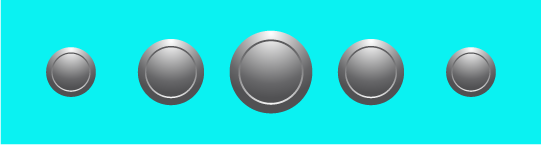# How do I create a magnifying scroll rect?

I have been able to create a scroll rect where the icons disappear at the edge of the scrolling area. However, I cannot find any direction on how to get my icons to magnify in scale the closer to the center of the scrolling area it gets. Any help would be greatly appreciated.

The following image should illustrate the effect I am going for:Can you get the distance from the center of your scrolling area to the position of the icon? You could use that to determine a scale multiplier.

Get the distance of the icon from the center and divide that by the total distance from the edge of the scroll rect to its center. That will give you a percentage value that represents how close to the center the icon is.
You can then set the localscale of the icon using this percentage value.

``````// The total width of the scroll rect
float rectWidth = 1000f;
// How far from the edge the center is: 500f
float rectCenter = rectWidth / 2f;

// The x position within the scroll rect
float iconPosX = 200f;
// The distance to the center: 300f
float iconFromCenter = rectCenter - iconPosX;

// a percentage value that represents the percentage from the
// edge to the center that the icon is: 0.4f
float percentToCenter = 1 - (iconFromCenter / rectCenter);

icon.transform.localScale = new Vector3(percentToCenter, percentToCenter, percentToCenter);
``````

Now this formula by itself will cause your icon to completely scale from 0 to 1. It sounds like you won’t want that, you’ll probably want the scaling to go from 0.5f to 1f, and we can make that work as well.

``````// Represents the total percentage range that you want to scale within
float validRange = 0.5f;

// Calculate out the real scale value
float scaleValue = 1 - (validRange * percentToCenter);

icon.transform.localScale = new Vector3(scaleValue, scaleValue, scaleValue);
``````